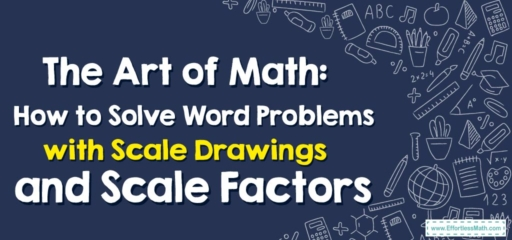# The Art of Math: How to Solve Word Problems with Scale Drawings and Scale Factors

Hello artful mathematicians!

Today we're going to combine the creativity of art class with the logic of math class as we navigate through word problems involving scale drawings and scale factors. Get ready to channel your inner artist and math whiz!## 1. The Art of Understanding: Scale Drawings and Scale Factors

The first brush strokes in our math-art masterpiece involve understanding the concept of scale drawings and scale factors:

• Scale Drawings: These are drawings that represent an object with accurate sizes reduced or enlarged by a certain amount.
• Scale Factors: This is the multiplier used to increase or decrease the dimensions of an object in a scale drawing.

## 2. The Canvas: Word Problems with Scale Drawings and Scale Factors

Now that we’ve mixed our paint (or math concepts), let’s start painting the picture with some word problems!

### Art Class Guide: Solving Word Problems with Scale Drawings and Scale Factors

#### Step 1: Decode the Problem

Identify what the problem is asking. Are we looking for an actual dimension, a drawing dimension, or a scale factor?

#### Step 2: Set Up the Scale

Determine the scale of the drawing. This is usually given in the problem or can be found by comparing the drawing and actual dimensions.

#### Step 3: Paint the Solution

Finally, use the scale to solve for the unknown. This usually involves some multiplication or division.

For example, suppose we have a scale drawing of a garden where $$1\ inch$$ represents $$4\ feet$$. If the drawing shows a path that is $$3\ inches$$ long, how long is the actual path?

1. Decode the Problem: We’re looking for the actual length of the path.
2. Set Up the Scale: $$1\ inch$$ on the drawing equals $$4\ feet$$ in real life.
3. Paint the Solution: Multiply the drawing length by the scale factor. So, $$3\ inches\times 4 \frac{feet}{inch} = 12\ feet$$. The actual path is $$12\ feet$$ long.

And voila! You’ve created a masterpiece by solving word problems involving scale drawings and scale factors. Remember, when art meets math, the possibilities are endless. Until our next creative math adventure, keep painting those numbers!

### What people say about "The Art of Math: How to Solve Word Problems with Scale Drawings and Scale Factors - Effortless Math: We Help Students Learn to LOVE Mathematics"?

No one replied yet.

X
30% OFF

Limited time only!

Save Over 30%

SAVE $5 It was$16.99 now it is \$11.99